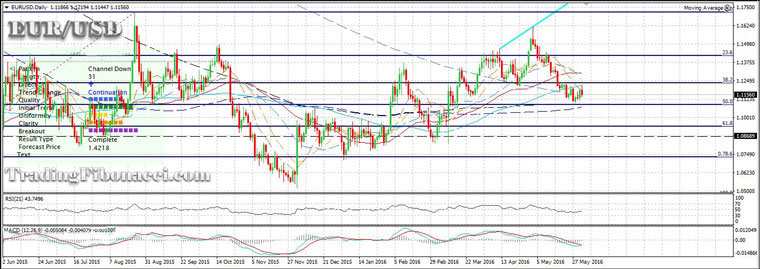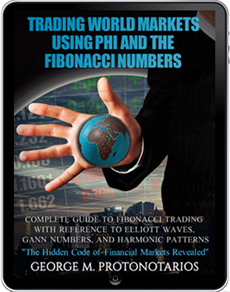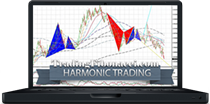Combining Fibonacci with Major Technical Analysis Tools

The Fibonacci tools can be used for multiple purposes. They can indicate support and resistance areas for trading the price breakouts and reversals, but also they can be used for achieving the optimal take-profit and stop-loss placements.

The Fibonacci Retracement tool can play a key role in many breakout trading strategies. Any trading signal generated by other technical analysis tools can be confirmed using the Fibonacci Ratios and their PRZ. In addition, a stop-loss order can be more reliable and more accurate if it is placed above or below major Fibonacci support or resistance zones. The Fibonacci level can be combined perfectly with momentum oscillators and breakout indicators.

The two key Fibonacci ratios are 38.2% and 61.8%. When a price breakout finally occurs, usually the price will move significantly outside of the 38.2% and 61.8% ratios.

Combining the Fibonacci Retracement with MACD

The MACD is one of the most widely used technical analysis oscillators and can confirm almost perfectly the Fibonacci Retracement levels. The MACD actually indicates the relationship between two moving averages.

MACD can be used for indicating crossovers, divergences or strong trends.

Using MACD (12, 26, 9)

If you select MACD on its default settings (12, 26, 9) focus on three-time frames (H1, H4, and D1) where MACD is significantly reliable.

-When the MACD crosses above the signal line, a bullish signal is confirmed

-When the MACD falls below the signal line, a bearish signal is confirmed

-Search also for divergences between the slope of the MACD and the slope of the Price. These divergences are indicating price exhaustion.

Chart: Fibonacci Levels and MACDCombining the Fibonacci Retracement with RSI

You may also use RSI for confirming trend reversals. The default number of RSI periods is 14 periods, RSI (14). But alternatively, you can use the 21 periods (21 is a Fibonacci number).

RSI (21) is more accurate than RSI (14):

-For weekly swings, use RSI (21) in the D1 time frame

-For daily swings, use RSI (21) in the M5 timeframe

Indications:

(i) RSI at 30 signifies oversold levels (confirming potential trend reversals for long trades)

(ii) RSI at 70 signifies overbought levels (confirming potential trend reversals for opening short trades).

(iii) Search also for divergences between the RSI slope and the price chart slope. As in the case of MACD, these divergences signify that the trend is exhausting.

Combining the Fibonacci Retracement with Bollinger Bands

Bollinger Bands is a powerful breakout indicator. If the price has crossed the upper or lower Bollinger Band at the same time when key Fibonacci Levels have been crossed, then the likelihood of a breakout is confirmed.

On the other hand, if the price has reversed after touching the upper or lower Bollinger Band and at the same time when key Fibonacci Levels have been reached, then the likelihood of a reversal is confirmed.

Combining the Fibonacci Retracement with Price Patterns

The Fibonacci Ratios can be combined with any other technical analysis tool, including price patterns. In the case of a Head & Shoulders pattern, the second (right) shoulder usually converges to 61.8% or 78.6% Fibonacci retracement level.

Combining the Fibonacci Retracement with Moving Averages

The Fibonacci Ratios can be combined also with Moving Averages. There are many types of Moving Averages and they can be used in almost any timeframe. Therefore, keeping things simple is the key.

Popular Types of Moving Averages

1. Simple Moving Average (SMA)

2. Exponential Moving Average (EMA)

3. Weighted Moving Average (WMA)

You can use the Fibonacci Numbers as the number of any Moving Average's periods.

M.A. Periods: 5, 8, 13, 21, 34, 55, 89, 144, 233, 377, 610, etc.

The Fibonacci numbers can be applied on SMAs, EMAs, WMAs

The Fibonacci MAs can be used in any time frame (from 5-Minute to 1-Day).

Tips:

(1) In shorter time frames, longer Fibonacci MAs are more reliable. For example, if you apply the 5-Period SMA on the M5 timeframe the results are basically irrelevant. In these shorter time frames, you should focus on 55-Period or higher MAs.

(2) On the other hand, in longer timeframes (H4, D1) you can use shorter MAs (i.e. 5, 8, 13).

(3) In most time frames, focus on the price crossovers above or below MA(55), MA(144), or MA(233)

Combining the Fibonacci Retracement with Major Technical Analysis Tools

Compare Online Brokers: » Compare ECN / STP Brokers

### Online Forex BrokersFibonacci mathematics aims to reveal the hidden proportionality of market behavior. Find Fibonacci trading tools and tutorials:

eBOOKTRADING WORLD MARKETS USING PHI AND THE FIBONACCI NUMBERS (2018)

Complete Guide to Fibonacci Trading with Reference to Elliott Waves, Gann Numbers, and Harmonic Patterns### Chart PatternsIdentify and Trade Reliable Chart Patterns:

» Elliott Wave Patterns

» Candlestick Formations